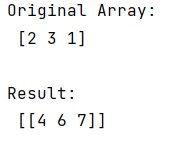# How can I 'zip sort' parallel numpy arrays?

Learn, how to 'zip sort' parallel numpy arrays in Python?
Submitted by Pranit Sharma, on January 19, 2023

NumPy is an abbreviated form of Numerical Python. It is used for different types of scientific operations in python. Numpy is a vast library in python which is used for almost every kind of scientific or mathematical operation. It is itself an array which is a collection of various methods and functions for processing the arrays.

Suppose we have two parallel lists and we want to sort them by the order of the elements in the first.

We need to sort them using NumPy arrays without unpacking them into conventional Python lists. For this purpose, we first need to find a permutation that sorts the array. We can use argsort for this.

Now we will apply the same permutation on the other array and store the result in B.

Let us understand with the help of an example,

## Python code to 'zip sort' parallel numpy arrays

```# Import numpy
import numpy as np

# Creating an array
arr = np.array([2, 3, 1])

# Display original array
print("Original Array:\n",arr,"\n")

# Sorting array
res = np.random.shuffle(arr)

# Applying same permutation
# on the other array
B = np.array([4, 6, 7])

# Getting result
res = B[res]

# Display result
print("Result:\n",res)
```

Output:What's New (MCQs)

Top Interview Coding Problems/Challenges!

IncludeHelp's Blogs

Languages: » C » C++ » C++ STL » Java » Data Structure » C#.Net » Android » Kotlin » SQL
Web Technologies: » PHP » Python » JavaScript » CSS » Ajax » Node.js » Web programming/HTML
Solved programs: » C » C++ » DS » Java » C#
Aptitude que. & ans.: » C » C++ » Java » DBMS
Interview que. & ans.: » C » Embedded C » Java » SEO » HR
CS Subjects: » CS Basics » O.S. » Networks » DBMS » Embedded Systems » Cloud Computing
» Machine learning » CS Organizations » Linux » DOS
More: » Articles » Puzzles » News/Updates